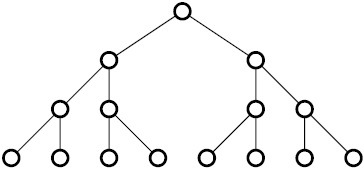# Path Length in a Binary Tree

Probability Level 4

A full binary tree of height 4 has 15 nodes, as pictured below.The 8 nodes at the bottom of the tree are called end nodes or leaf nodes. Two distinct end nodes are uniformly chosen. The expected length of the shortest path between them can be expressed as $\frac{a}{b}$, where $a$ and $b$ are coprime positive integers. What is the value of $a + b$?

×

Problem Loading...

Note Loading...

Set Loading...